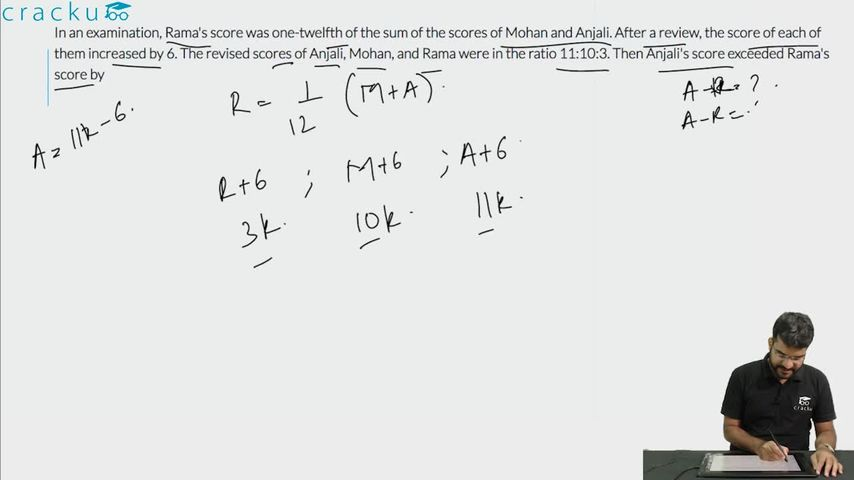Question 100

# In an examination, Rama's score was one-twelfth of the sum of the scores of Mohan and Anjali. After a review, the score of each of them increased by 6. The revised scores of Anjali, Mohan, and Rama were in the ratio 11:10:3. Then Anjali's score exceeded Rama's score by

Solution

Let the scores of Rama, Anjali and Mohan be r, a, m.

It is given that Rama's score was one-twelfth of the sum of the scores of Mohan and Anjali

r=$$\ \frac{\ m+a}{12}$$ ———-(1)

The scores of Rama, Anjali and Mohan after review = r+6, a+6, m+6

a+6:m+6:r+6 = 11:10:3

Let a+6 = 11x => a= 11x-6

m+6=10x => m=10x-6

r+ 6 =3x => r = 3x-6
Substituting these values in equation (1), we get

3x-6=$$\ \frac{\ 21x-12}{12}$$

12(3x-6) = 21x-12

x=4

Anjali's score exceeds Rama's score by (a-r)=8x=32

### View Video Solution